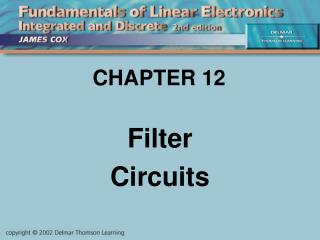DownloadDownload PresentationCHAPTER 12

CHAPTER 12

Télécharger la présentationCHAPTER 12

- - - - - - - - - - - - - - - - - - - - - - - - - - - E N D - - - - - - - - - - - - - - - - - - - - - - - - - - -
Presentation Transcript

1. CHAPTER 12 Filter Circuits

2. Objectives Describe and Analyze: • Filter types: LPF, HPF, BPF, BSF • Passive filters • Active filters • LC tuned amplifiers • Other filter topics • Troubleshooting

3. Introduction A filter is a circuit designed to separate signals from each other based on their frequency. There are four basic types: • Low-Pass Filter (LPF): passes signals below some frequency • High-Pass Filter (HPF): passes signals above some frequency • Bandpass Filter (BPF): passes signals between two frequencies • Bandstop Filter (BSF): blocks signals between two frequencies

4. Frequency Response Bands are measured to the 3dB points.

5. Passive RC Filters • All four types of filter can be made with just resistors and capacitors. They are not “high performance”, but they work.

6. RC Filters The frequency f = 1/(2RC) has several names: • Break frequency • Corner frequency • Cutoff frequency • Roll-off frequency • 3dB frequency (or -3dB frequency) • 0.707 frequency • f0 (“eff-zero”) • fB (“eff-bee”)

7. RC Filters Bode plot for a”single-stage” LPF (one R and one C)

8. RC LPF Example What is the cutoff frequency for a LPF with R = 1.59 k and C = 0.01 F ? Time constant  = RC = (1.59 k)  (0.01 F) = 15.9 s f0 = 1 / (2) = 10 kHz

9. Bode Plots Note that 6 dB /octave is equal to 20 dB /decade.

10. Order • The term order used to describe filters tells us how fast the Bode plot rolls off. A first-order filter, such as an RC filter made with one capacitor, has a roll-off of 20 dB/decade, a second-order filter has a roll-off of 40 dB/decade, and so on. • The roll-off is N 20 dB/decade where N is the order of the filter. • You will see the word pole used to mean the same thing: a 1-pole filter is a first-order filter, a 2-pole filter is a second-order filter, and so on.

11. Order <insert figure 12-10 here> The Bode plot says it all.

12. Active Filters • When you make a filter, you want its Bode plot to have a shape appropriate to the application. While a second-order filter can be made with two resistors and two capacitors, its Bode plot will not have a “clean” break-point. That’s where active filters come in. • Active filters use an op-amp together with Rs and Cs. The op-amp’s feedback loop allows you to control the shape of the Bode plot. • Feedback RC filters are often called “Sallen & Key” filters after the two men who first described them in the 1950s. Of course, they used vacuum tubes!

13. Active Filters (a) is a LPF, (b) is a HPF

14. Active Filters <insert figure 12-16 here>

15. Active Filters

16. Switched Capacitor Filters Break-point is controlled by clock rate.

17. LC Tuned Amplifier

18. LC Tuned Amps andQ • A tuned circuit amplifier is essentially a bandpass filter with a very narrow pass band. The parameter Q (stands for “Quality”) measures the narrowness of the pass band. How high is high depends on the application, but usually Q = 10 or more is high Q. The width of the pass band is the center frequency divided by Q. • Q = REQ /(2 foL) where REQ is the equivalent resistance across the parallel LC circuit.

19. Piezoelectric Filters Piezoelectric crystals and ceramics act like tuned circuits.

20. Troubleshooting • Determine the filter type you are working on. • Use an oscilloscope to look at inputs and outputs to check for correct filter response. • If necessary, inject a sine wave and vary the frequency to test the filter response. • Tuned-circuits sometimes “drift”, and may have a small trimmer capacitor to make adjustments. • Active filters either work or the op-amp is dead. • Crystals and ceramics do not drift, but they can crack from rough handling or too much current.